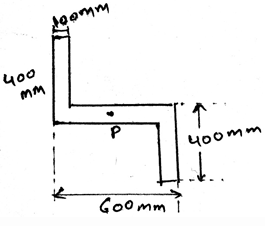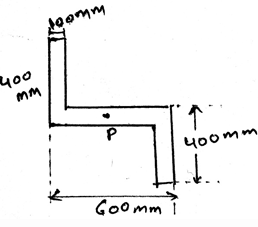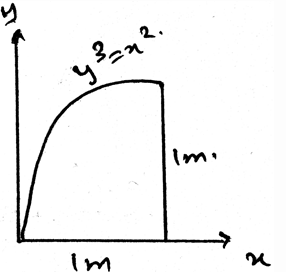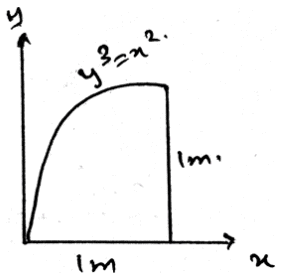# Test: Moments Of Inertia For An Area About Inclined Axis

## 30 Questions MCQ Test Engineering Mechanics - Notes, Videos, MCQs & PPTs | Test: Moments Of Inertia For An Area About Inclined Axis

Description
This mock test of Test: Moments Of Inertia For An Area About Inclined Axis for Mechanical Engineering helps you for every Mechanical Engineering entrance exam. This contains 30 Multiple Choice Questions for Mechanical Engineering Test: Moments Of Inertia For An Area About Inclined Axis (mcq) to study with solutions a complete question bank. The solved questions answers in this Test: Moments Of Inertia For An Area About Inclined Axis quiz give you a good mix of easy questions and tough questions. Mechanical Engineering students definitely take this Test: Moments Of Inertia For An Area About Inclined Axis exercise for a better result in the exam. You can find other Test: Moments Of Inertia For An Area About Inclined Axis extra questions, long questions & short questions for Mechanical Engineering on EduRev as well by searching above.
QUESTION: 1

### If the non-Uniform loading is of the type of parabola then for calculating the moment of inertia for areas inclined at an axis?

Solution:

The net force will act at the centroid of the parabola. Whether it be a parabola or the cubic curve the centroid is the only point at which the net force act. Force can’t be acted horizontally if the loading is vertical. Hence whatever be the shape of the loading, the centroid is the point of action of net force. Thus the use of centroid.

QUESTION: 2

### Determine the orientation of the principle axis for the cross section of area of member shown whose width is 100mm.Solution:

The net force will act at the centroid of the parabola. Whether it be a parabola or the cubic curve the centroid is the only point at which the net force act. Force can’t be acted horizontally if the loading is vertical. Hence whatever be the shape of the loading, the centroid is the point of action of net force. Thus the use of centroid.

QUESTION: 3

### The product of Inertia for an area is required so as to__________

Solution:

The product is required as to know what is the maximum and the minimum moment of inertia of an area. This data is required as to design the structure of the body. This means that the designing of the body is majorly done by the help of the determination of the product of the moment of Inertia.

QUESTION: 4

Moment of Inertia about an inclined axis is the integration of the square of the distance of the centroid and the del area along the whole area of the structure and after this calculations we multiply the moment of areas.

Solution:

The moment of inertia of the section is the integration of the square of the distance of the centroid and the del area along the whole area of the structure. This is having much significance in the various fields in the engineering sector. The main types are the ‘I’ section structures which are being much used.

QUESTION: 5

The product of moment of inertia is the sum of _____________ and _________________. It can be used in the calculations of moments of inertia for an area about inclined axis.

Solution:

The product of moment of inertia is required as to design the structure of the body. This means that the designing of the body is majorly done by the help of the determination of the product of the moment of Inertia.

QUESTION: 6

There is perpendicular axis theorem for the area, and it is can be used to determine the moment of inertia of an area about inclined axis.

Solution:

There is no perpendicular axis theorem for the area. In spite there is the theorem as parallel axis for any area. Thus we have the theorem which is used to add the two mutually perpendicular moment of inertias.

QUESTION: 7

If any external force also is applied on the structure and we are determining the moment of inertia for areas about inclined axis then what should we consider?

Solution:

The external forces are treated differently. They are not added by the force of the distributed loading. That is the force not only acts at the centroid always. It can be shifted also. Depending on the external forces. Thus the use of centroid or centre of mass.

QUESTION: 8

Determine the magnitude of the principle moment of inertia for the cross section of area of member shown whose width is 100mm.Solution:

The net force will act at the centroid of the parabola. Whether it be a parabola or the cubic curve the centroid is the only point at which the net force act. Force can’t be acted horizontally if the loading is vertical. Hence whatever be the shape of the loading, the centroid is the point of action of net force. Thus the use of centroid.

QUESTION: 9

The calculation of moment of inertia about inclined axis due to the loadings involve a quantity called ____________

Solution:

The calculation of the moment of the body due to the loadings involve a quantity called moment of inertia. This is having much significance in the various fields in the engineering sector. The main types are the ‘I’ section structures which are being much used.

QUESTION: 10

Whenever the distributed loading acts perpendicular to an area its intensity varies __________ for the determination of moment of inertia about the inclined axis.

Solution:

The load intensity is varying linearly in the structures. Thus the intensity is not varying parabolically nor is it cubically. It cannot be a vector also. Thus the intensity is linearly varied.

QUESTION: 11

The distance in the parallel axis theorem for the use in the determination of the moment of inertia about an inclined axis is multiplied by:

Solution:

Parallel axis for any area is used to add the two mutually perpendicular moment of inertias for areas. It gives a moment of inertia perpendicular to the surface of the body. And uses the square of the distance from the axis of rotation multiplied by the area.

QUESTION: 12

One of the use of the centre of mass or centroid is as in the determination of the moment of inertia about an inclined plane is that the net force acts at the ___________ of the loading body.

Solution:

In the moment of inertia calculations we see that the net force acts at the centroid of the loading body. That is if the loading system is in the form of the triangle then the at the distance 2 by 3 of the base the net force of the loading will act. And the load will be half the area of the loading.

QUESTION: 13

What is parallel axis theorem and to whom it is applied so that it can give the product of inertia of an area of an area inclined about an axis?

Solution:

Parallel axis for any area is used to add the two mutually perpendicular moment of inertias for areas. It gives a moment of inertia perpendicular to the surface of the body. That is the moment of inertia perpendicular to the surface in considerance.

QUESTION: 14

The body is sometimes acted by two or three force members and we need to find the moment of inertia about the inclined axis for the same. The difference between the two and the three force members is:

Solution:

The definition of the two force member only defines that the forces are being acted on the two points on the body. So does is the definition of the three forces members. The points of action of the three forces are three.

QUESTION: 15

The parallel axis theorem can add any angle varied moment of inertias to give the perpendicular moment of inertia and it can be used in the determination of the moment of inertia about inclined axis.

Solution:

Parallel axis for any area is used to add the two mutually perpendicular moment of inertias for areas. It gives a moment of inertia perpendicular to the surface of the body. That is the moment of inertia perpendicular to the surface in considerance.

QUESTION: 16

In the calculations of the moment of inertia of the area about an inclined axis, we have some transformation done. They are:

Solution:

In the moment of inertia calculations we see that the net force acts at the centroid of the loading body. That is if the loading system is in the form of the triangle then at the distance 2 by 3 of the base the net force of the loading will act. And the load will be half the area of the loading. Thus when there is the inclination of the axis, we use transformation.

QUESTION: 17

In the calculations of moments of inertia for an area about inclined axis we use the product of moment of inertia. It is the the sum of _____________ and _________________

Solution:

The product of moment of inertia is required as to design the structure of the body. This means that the designing of the body is majorly done by the help of the determination of the product of the moment of Inertia.

QUESTION: 18

In the calculations of the moment of inertia of the area about an inclined axis, we have some transformation done. They are:

Solution:

In the moment of inertia calculations we see that the net force acts at the centroid of the loading body. That is if the loading system is in the form of the triangle then at the distance 2 by 3 of the base the net force of the loading will act. And the load will be half the area of the loading. Thus when there is the inclination of the axis, we use transformation.

QUESTION: 19

In the calculations of the moment of inertia of the area about an inclined axis, we have some transformation done. They are:

Solution:

In the moment of inertia calculations we see that the net force acts at the centroid of the loading body. That is if the loading system is in the form of the triangle then at the distance 2 by 3 of the base the net force of the loading will act. And the load will be half the area of the loading. Thus when there is the inclination of the axis, we use transformation.

QUESTION: 20

In the calculations of the moment of inertia of the area about an inclined axis, we have some transformation done. They are:

Solution:

In the moment of inertia calculations we see that the net force acts at the centroid of the loading body. That is if the loading system is in the form of the triangle then at the distance 2 by 3 of the base the net force of the loading will act. And the load will be half the area of the loading. Thus when there is the inclination of the axis, we use transformation.

QUESTION: 21

There is parallel axis theorem for the area, and it is can be used to determine the moment of inertia of an area about inclined axis.

Solution:

There is no perpendicular axis theorem for the area. In spite there is the theorem as parallel axis for any area. Thus we have the theorem which is used to add the two mutually perpendicular moment of inertias.

QUESTION: 22

Determine the moment of inertia of the area about y-axis.Solution:

Parallel axis for any area is used to add the two mutually perpendicular moment of inertias for areas. It gives a moment of inertia perpendicular to the surface of the body. That is the moment of inertia perpendicular to the surface in considerance.

QUESTION: 23

In the calculations of the moment of inertia of the area about an inclined axis, we have some transformation done. They are:

Solution:

In the moment of inertia calculations we see that the net force acts at the centroid of the loading body. That is if the loading system is in the form of the triangle then at the distance 2 by 3 of the base the net force of the loading will act. And the load will be half the area of the loading. Thus when there is the inclination of the axis, we use transformation.

QUESTION: 24

In the calculations of the moment of inertia of the area about an inclined axis, we have some transformation done. They are:

Solution:

In the moment of inertia calculations we see that the net force acts at the centroid of the loading body. That is if the loading system is in the form of the triangle then at the distance 2 by 3 of the base the net force of the loading will act. And the load will be half the area of the loading. Thus when there is the inclination of the axis, we use transformation.

QUESTION: 25

Moment of Inertia about an inclined axis is the integration of the cube of the distance of the centroid and the del area along the whole area of the structure and after this calculations we multiply the moment of areas.

Solution:

The moment of inertia of the section is the integration of the square of the distance of the centroid and the del area along the whole area of the structure. This is having much significance in the various fields in the engineering sector. The main types are the ‘I’ section structures which are being much used.

QUESTION: 26

Determine the moment of inertia of the area about x-axis.Solution:

Parallel axis for any area is used to add the two mutually perpendicular moment of inertias for areas. It gives a moment of inertia perpendicular to the surface of the body. That is the moment of inertia perpendicular to the surface in considerance.

QUESTION: 27

In the calculations of the moment of inertia of the area about an inclined axis, we have some transformation done. They are:

Solution:

In the moment of inertia calculations we see that the net force acts at the centroid of the loading body. That is if the loading system is in the form of the triangle then at the distance 2 by 3 of the base the net force of the loading will act. And the load will be half the area of the loading. Thus when there is the inclination of the axis, we use transformation.

QUESTION: 28

In the calculations of the moment of inertia of the area about an inclined axis, we have some transformation done. They are:

Solution:

In the moment of inertia calculations we see that the net force acts at the centroid of the loading body. That is if the loading system is in the form of the triangle then at the distance 2 by 3 of the base the net force of the loading will act. And the load will be half the area of the loading. Thus when there is the inclination of the axis, we use transformation.

QUESTION: 29

In the calculations of the moment of inertia of the area about an inclined axis, we have some transformation done. They are:

Solution:

In the moment of inertia calculations we see that the net force acts at the centroid of the loading body. That is if the loading system is in the form of the triangle then at the distance 2 by 3 of the base the net force of the loading will act. And the load will be half the area of the loading. Thus when there is the inclination of the axis, we use transformation.

QUESTION: 30

If any external moment along with the force is applied on the structure and we are determining the moment of inertia for areas about inclined axis then what should we consider?

Solution:

The external forces are treated differently. They are not added by the force of the distributed loading. That is the force not only acts at the centroid always. It can be shifted also. Depending on the external forces. Thus the use of centroid or centre of mass.